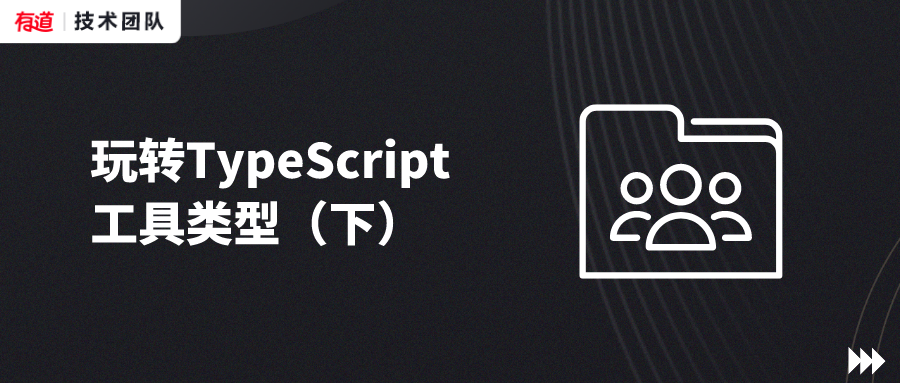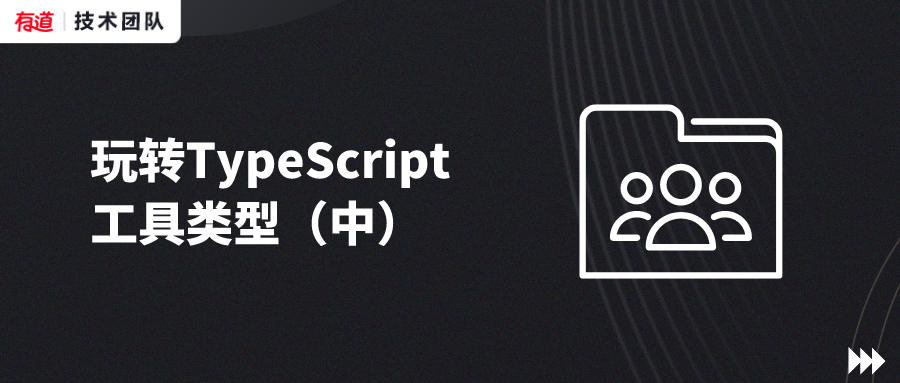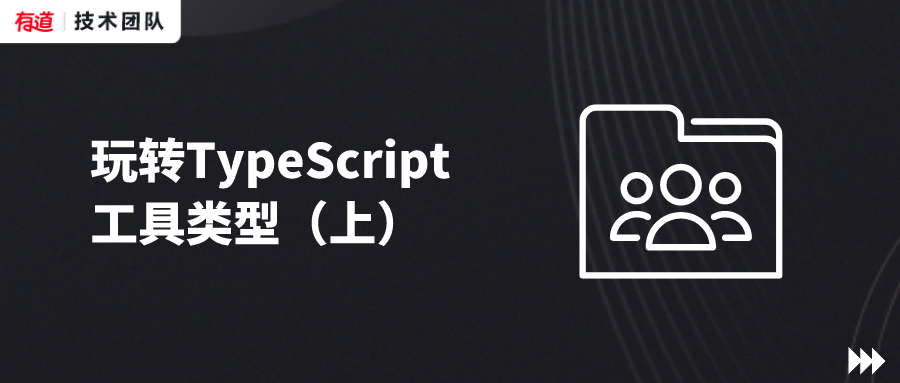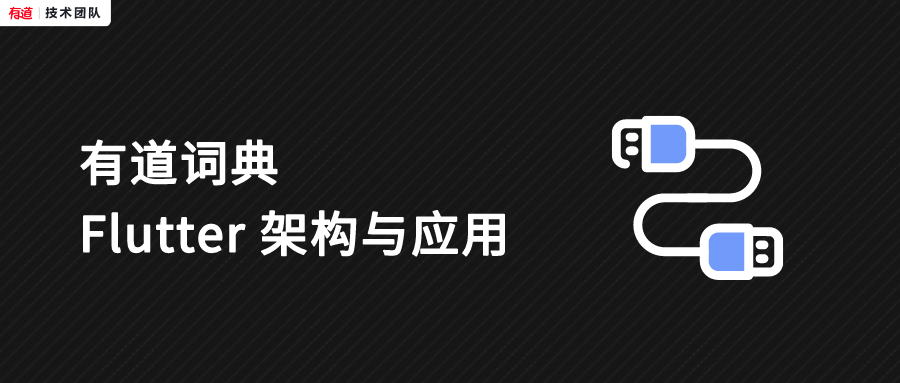# 玩转TypeScript工具类型（下）

2021/09/22 18:30• ThisParameterType<Type>

• OmitThisParameter<Type>

• ThisType<Type>

youdao

ydtech

• this 参数 只能叫 this，且必须在参数列表的第一个位置；
• this 必须是 显式定义的；
• 这个 this 参数在函数实际被调用的时候不存在，不需要显式作为参数传入，而是通过 call apply 或者是 bind 等方法指定。

1.1

type ThisParameterType<T> = T extends (this: infer U, ...args: any[]) => any ? U : unknown;

1.2

function toHex(this: Number) {  return this.toString(16);} function numberToString(n: ThisParameterType<typeof toHex>) {  return toHex.apply(n);}

youdao

ydtech

2.1

type OmitThisParameter<T> = unknown extends ThisParameterType<T> ? T : T extends (...args: infer A) => infer R ? (...args: A) => R : T;

unknown extends ThisParameterType<T> ? T : ...

T extends (...args: infer A) => infer R ? (...args: A) => R : T

2.2

function toHex(this: Number) {  return this.toString(16);} const fiveToHex: OmitThisParameter<typeof toHex> = toHex.bind(5); console.log(fiveToHex());

youdao

ydtech

/** * Marker for contextual 'this' type */interface ThisType<T> { }

let demo1 = {  a: 'lipengpeng',  test(msg: string) {    this;  }};

this: {    a: string;    test(msg: string): void;}

let demo2 = {  a: 'lipengpeng',  test(this:{a: string}, msg: string) {    this;  }};

this: {    a: string}

let options = {  data: {    x: 0,    y: 0  },  methods: {    moveBy(dx: number, dy: number) {      this.x += dx;      this.y += dy;    }  }}

function makeObject(config) {  let data = config?.data || {}  let methods = config?.methods || {}  return {    ...data,    ...methods  }}let options = makeObject({  data: {    x: 0,    y: 0  },  methods: {    moveBy(dx: number, dy: number) {      this.x += dx;      this.y += dy;    }  }})

// 只考虑传入makeObject的config参数只包含data和methods两个参数// 定义两个泛型参数D & M来代表它们的类型type ObjectConfigDesc<D, M> = {  data: D  methods: M}function makeObject<D, M>(config: ObjectConfigDesc<D, M>): D & M {  let data = config?.data || {}  let methods = config?.methods || {}  return {    ...data,    ...methods  } as D & M}

type ObjectConfigDesc<D, M> = {  data: D  methods: M & ThisType<D & M>}

let options: {    x: number;    y: number;} & {    moveBy(dx: number, dy: number): void;}

youdao

ydtech### 作者的其它热门文章

0
3 收藏

0 评论
3 收藏
0